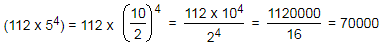# Number System - Online Test

Q1. When you reverse the digits of the number 13, the number increases by 18. How many other two digit numbers increase by 18 when their digits are reversed?
Explaination / Solution:

Let the number be (10x + y), so when the digits of number are reversed the number becomes (10y + x).

According the question, (10y + x) – (10x + y) = 18

⇒ 9 (y – x) = 18           ⇒y – x = 2

So, the possible pairs of (x, y) are

(1, 3), (2, 4), (3, 5), (4, 6), (5,7), (6, 8) and (7,9).

But we want the number other than 13.

Thus, there are 6 possible numbers, i.e., 24, 35, 46, 57, 68, 79.

So, total number of possible numbers are 6.

Q2. A rectangular floor is fully covered with square tiles of identical size. The tiles on the edges are white and the tiles in the interior are red. The number of white tiles is the same as the number of red tiles. A possible value of the number of tiles along one edge of the floor is :
Explaination / Solution:

Let the rectangle has x and y tiles along its length and breadth respectively.

The number of white tiles

W = 2x + 2(y – 2) = 2 (x + y – 2)

And the number of red tiles = R = xy – 2 (x + – 2)

Given that the number of white tiles is the same as the number of red tiles

⇒ 2 (x + y – 2) = xy – 2 (x + – 2)

⇒ 4 (x + y – 2) = xy

⇒ xy – 4x – 4y = –8

⇒ (x – 4) (y – 4) = 8 = 8 ×1 or 4 × 2

m – 4 = 8 or 4 ⇒= 12 or 8

Therefore.the number of tiles along one edge of the floor can be 12

Q3. X persons stand on the circumference of a circle at distinct points. Each possible pair of persons, not standing next to each other, sings a two-minute song one pair after the other. If the total time taken for singing is 28 minutes, what is X?
Q4. Each family in a locality has at most two adults, and no family has fewer than 3 children. Considering all the families together, there are more adults than boys, more boys than girls, and more girls than families. Then the minimum possible number of families in the locality is :
Explaination / Solution:

Given, Number of adults > Number of boys > Number of girls > Number of families.

Going back from the choices, let us start with the least value given in the choices.

Since the minimum possible number of families has been asked.

In choice (c), Number of families = 2

⇒Number of girls > 3, Number of boys > 4 and Number of adults > 5

But two families together can have a maximum of 4 adults.

Therefore, the number of families is not equal to 2.

In Choice (d). Number of families = 3.

Therefore, the Number of (girls) > 4. Number of (boys) > 5 and Number of (adults) > 6

Q5. The owner of a local jewellery store hired 3 watchmen to guard his diamonds, but a thief still got in and stole some diamonds. On the way out, the thief met each watchman, one at a time. To each he gave 1/2 of the diamonds had then, and 2 more besides. He escaped with one diamond. How many did he steel originally?
Q6. Which one of the following is not a prime number?
Explaination / Solution:
No Explaination.

Q7. (112 x 54) = ?
Explaination / Solution:Q8. It is being given that (232 + 1) is completely divisible by a whole number. Which of the following numbers is completely divisible by this number?
Explaination / Solution:

Let 232 = x. Then, (232 + 1) = (x + 1).

Let (x + 1) be completely divisible by the natural number N. Then,

(296 + 1) = [(232)3 + 1] = (x3 + 1) = (x + 1)(x2 - x + 1), which is completely divisible by N, since (x + 1) is divisible by N.

Q9. What least number must be added to 1056, so that the sum is completely divisible by 23 ?
Explaination / Solution:

```23) 1056 (45
92
---
136
115
---
21
---

Required number = (23 - 21)
= 2.```

Q10. 1397 x 1397 = ?
Explaination / Solution:

 1397 x 1397 = (1397)2 = (1400 - 3)2 = (1400)2 + (3)2 - (2 x 1400 x 3) = 1960000 + 9 - 8400 = 1960009 - 8400 = 1951609.